IB - Casio Calculators

## IB## Students & Parents

Maths is compulsory (both SL and HL) for the full IB Diploma. This reflects the prevalence of mathematics in our lives, with all its interdisciplinary connections. Students are expected to have access to a graphic calculator at all times during the course and in exams.

You’ll be learning areas of maths you’ve not yet encountered. There will be new and more complex types of calculation. You’ll explore and analyse algebraic graphs. If you study Further Maths you’ll discover entirely new types of number and graph. You need a good quality graphic calculator. We recommend the fx-CG50 graphic calculator.

## What does a graphic calculator do?

Graphical calculators don’t just display graphs; they allow you to interact with them.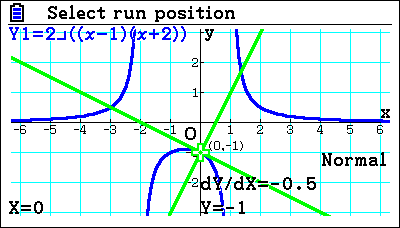You can undertake complex calculations that are not possible on a scientific calculator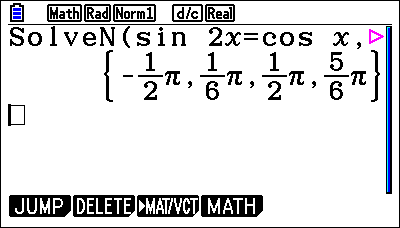There is a wide range of statistical and probability functions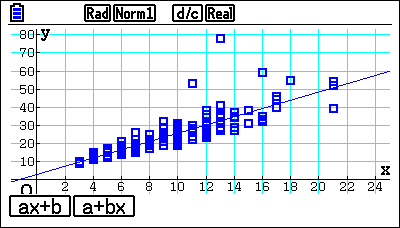## Related Resources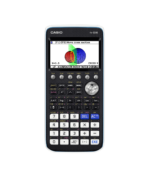How to Video
fx-CG50 Further Maths IB
Complex Numbers – Calculations and Equations

How to enter calculations for complex numbers. Solve the complex roots of polyno…How to Video
fx-CG50 A-Level GCSE IB
Quick Skill: Calculations Using Coordinate Values

How to extract coordinate values from points of interest in Graph to perform ope…How to Leaflet
fx-CG50 A-Level GCSE IB
Run-Matrix Quick Start fx-CG50

How to enter basic calculations on the fx-CG50. Includes using ANS for simple it…How to Video
fx-CG50 A-Level IB
Integration – Mixed

How to integrate graphically and numerically between two functions using interse…How to Video
fx-CG50 A-Level GCSE IB
Quick Skill: Solving Linear Equations

How to solve a linear equation using the numerical SolveN function….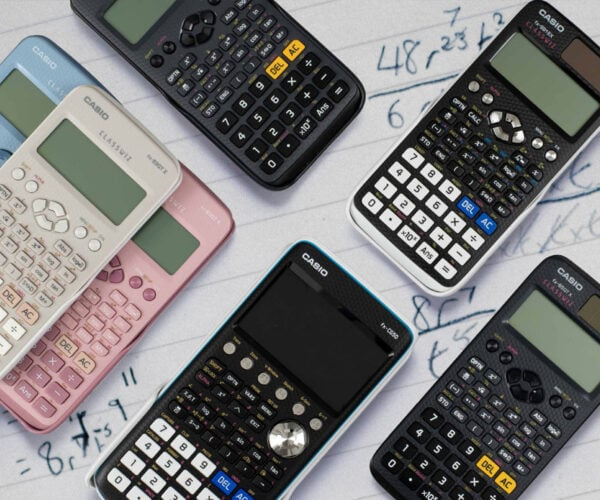### Calculator comparison

Casio offer a range of calculators for the large variety of maths, science and engineering courses across the United Kingdom and Ireland.### Learn how to Use the fx-CG50

Our extensive resources hub provides videos and pdfs, as well as ‘how to’ videos and downloads.### Examinations

All calculator models sold by Casio UK are permitted in UK school exams. Some models are permitted in Irish and IB exams.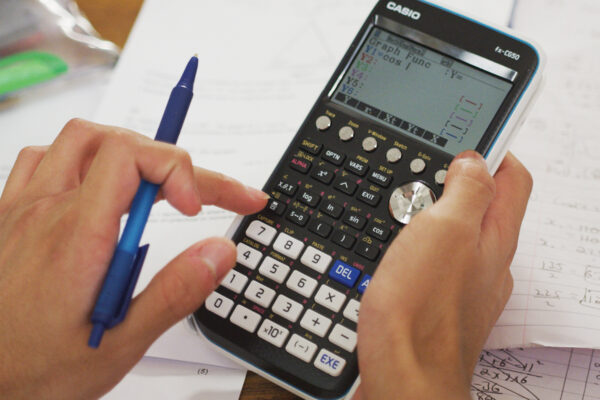## Should I Buy A Graphic Calculator?

Maths is a visual subject. A graphic calculator allows students to explore graphs. For example, you could plot a graph, find the coordinates where two graphs cross, or draw a data chart.

Graphic calculators make learning a lot easier and more fun. They also give an advantage in exams. We recommend the fx-CG50 calculator for more confident mathematicians, perhaps those taking the Higher tier. Our graphic calculators are allowed in GCSE exams. Explore the detailed specification of the calculator here.